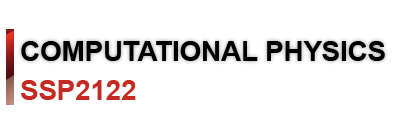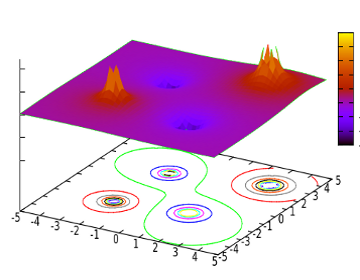## Topic outline

•### GeneralLecturers : Mohd Khalid Kasmin, Abd. Khamim Ismail : Physics Department, Faculty of Science. Semester : Sem I 2010/2011

Synopsis.

This is a course aims at removing some of the mysteries out of using a computer to do meaningful physical calculation. Students will be introduced to a number of basic methods on how to investigate common introductory physics problems using the fast calculator commonly known as computer.

Students will be taught basic computer programming using Java language in Linux or Windows environment.

Result of calculation will be printed to screen for immediate inspection. Large output then can easily be redirected to a file in the hard disk and using a command line visualization program the data in the file may be plotted onto graphic screen and the graph can be saved as an image in various file format.

Students will also be introduce to ways on how to do computation across computer network and how to visualize result of computation from remote machine on local machine.

Learning outcomes
On successful completion of this course, student should be able, at threshold level, to:
• formulate and culculate simple physics problems using computer.
• design a simple interface for interactive physics solver.
• develope a java program for solving elementary physics problem.This work, SSP2122 Computational Physics by Mohd Khalid Kasmin & Dr. Abd. Khamim Ismail is licensed under a Creative Commons Attribution-NonCommercial-ShareAlike 3.0 Unported License.

•### Topic 1

 01. IntroductionOne good description of computational physics - "is the study and implementation of numerical algorithms in order to solve problems in physics for which a quantitative theory already exists. It is often regarded as a subdiscipline of theoretical physics but some regard it as an intermediate branch of physics between theoretical and experimental. " http://en.wikipedia.org/wiki/Computational_physics 02. Working with LinuxLinux is an OS, an operating system, a program that manages how computation and communication is done in the system. Physically it is a small program, about 1 Mbytes in compressed form in the hard disk. In its simplest form, one can use the computer under linux via a command line interface (CLI) known as the linux console. In this mode however, we only have a very limited graphical capability.
•### Topic 2

 03. Survival kit for the command line environmentIntroduction to basic unix commands; ls, cd, cp, mkdir, rmdir, mv, and rm. 04. Login and working in remote Linux serverStudents is given an account (username with password) to log into a Linux server. One class hr is alloted to guide student to 1st time login and working in a remote server computer.

•### Topic 3

 05. Arithmatic calculation and display of outputUsing only System.out.println(), we begin looking at how java handles arithmatic calculation and how we can instruct it to display the result of a calculation. 06. Doing arithmatic calculation with the help of variablesThe real power of a computer program is its ability to handle many different variables for doing calculation.

•### Topic 4

 07. Repeatative calculation using for(;;) and display of formatted outputStudent is introduced to a way of doing iterative calculation, which the computer is excelent at, using the for(;;) loop. Previously we were using System.out.println() to get values out to the console, now we begin looking at how the output may be formatted using System.out.printf(). 08. How to do summationBeside iterative, much of physics calculations involve the computation of some resultant values. That means we need to do summation of physical quantities. So here we introduce the concept of array variable for storage of similar quantities, and show that by looping with respect to the indecies of the array we can do the needed summations.

•### Topic 5

 09. Resolving vector location and forceMost computer languages do not provide operators or building functions for addition, subtraction, multiplication, or division of vectors. So we need to know the technique on how to handle vector calculations since physics problems normally involve vector quantities. 10. Standard math libraryAddition, subtraction, multiplication, and division are not enough. There are times when we need to use some math functions like log(), sin(), cos(), etc. These functions are not in the basic language set of most major computer languages like C, Java, or C++. Hence students are introduce to the concept of importing external standard libraries or classes.

•### Topic 6

 11. Decision making structureUsing the if() statement when certain calculations need to be ignored or done under certain conditions. Introducing the comparison operators ==, !=, <=, >=, <, and > for the boolen statement in the argument of the if() . Using if()-else{} for cases of "if (true) do this else do that". Students are shown that the if()-else{} can be nested. 12. Nested loop - loop inside loopIt's been shown that summation can be computed with the help of for() loop. Sometimes there arise situation when we want to repeat a for() loop a number of times (with or without variation of some parameters). Here students are shown that we can put a for() loop inside another for() loop, namely construct nested loop.

•### Topic 7

 13. Array variables Array variable facilitates calculation involving large quantity of numbers representing common physical phenomena. Storage concept of arrayed data, declaration of array. 14. Example of usage of array variable.Calculation of garvitational force on an obejct due to many other neighboring objects. Array of m[ ] and r[ ]. Summations of F on m[ i ].

•### Topic 8

 15 Text based standard input/outputGetting input from standard input device the keyboard 16 Making an applicationNetbeans.GUI.Building a physics solver.

•### Topic 9

 17. Programming in IDEJava programming in an integrated development environment (IDE), NetBeans. Students are shown how to build a non-GUI Java program in NetBeans that works based on "project" concept. Where to locate the source code and the class file in the project folders. Distribution of Java project using jar file is made very easy using Netbeans, and students are shown where to locate the jar file and how to use it. 18. GUI programming in IDEBuilding a GUI based physics solver. Students are shown how to create a GUI interface for a simple physics solver. The GUI interface makes use of "Swing GUI Form" specifically the "JFrame Form". The swing controls introduced are the JLabel, JButton, and JTextField.

•### Topic 10

 19. GUI programming in IDE - Part 2After the GUI interface is ready, we need to make the application react properly to action acted upon it by the user. So, here students are introduces to the concept of event driven code activity: - How to attach event listener to a JButton, - how to read text from a JTextField, - how to convert the text to double value, - how to modify the text of a JLabel, - and how to exit properly when user click on the [Quit] button. 20. Solving ODE using Euler MethodMany physics problems are in the form of differential equation. Ability to handle such problem using computer programming is the central concern of computational physics. One classic problem in most beginning introductory physics is the Free falling body problem. Students are introduced to the Euler method (and the Euler algorithm) for solving the F = ma = m dv/dt = m d2y/dt2 .

•### Topic 11

 21. Improving Euler methodComparison between analytical solution of F=ma with the Euler method, shows that although the method is able to find approximate solution, the error is quite significant if computational step is reduced. The error is also cumulative, i.e as the iteration of the calculation increases the error also gets bigger, hence value furthest away from initial condition has the largest error.The Euler method gives us a way to approximate positions of object with respect to time, but requires an order of magnitude of computational iterations for each order of magnitude of accuracy. For large number of objects this method will certainly consume a great deal of computation time. Hence students are introduced to Initial Half-Step Method, an Euler method with a much improved computational effeciency. 22. Scanning for maximum/minimum point.In most computational physics work, we usually need to find maximum or minimum physical values. Student is introduced to common programming practice for scanning max/min or zero values while doing iteration.

•### Topic 12

 23. Projectile (two dimensional motion)The initial half-step method employed in the free falling body problem (1D problem) can also be used in 2D problem of projectile motion. 24. Restoring force F = -kx.Here we look at numerical solution of a simple harmonic motion, using the Euler method.

•### Topic 13

 25. Calculation of Electrostatic potential lines on X-Y plane.Using mesh method in calculation of V(r) and visualization of electrostatic potential surface using gnuplot. 26. Calculation of electrostatic potential for a group of point chargesYou will learn about - mesh method using a for() loop inside another for() loop to generate ordered pair (x,y) points in a mesh on x-y plane calculate physical value, in this case, V(x,y) for every point (x,y) generated - saving calculated values into a file making a sequential text file saving in a format that gnuplot can understand for making a surface plot - use gnuplot to visualize potential surface making a surface plot from data points in a text file making contour plot to visualize equipotential lines changing the z-axis range to zoom in or zoom out on the surface labelling the plot save as an image; the potential surface & the contour plot save as an eps file; the potential surface & the contour plot - include an eps file into a document

•### Topic 14

 27. Portability of java program.How to create a jar file. How to script code for running on web browser.